## Saturday, June 30, 2012

### Cubic Formula - BASIC Program (HP 71B)

Finding the roots of the cubic equation ax^3 + bx^2 + cx + d = 0 can be a challenge. Even with a cubic formula, multiple and sometimes complicated steps can be involved.

Source:

In this program my general strategy is:
1. Find the first root X1.
2. Use X1 to invoke synthetic division to reduce the cubic equation to a quadratic equation.
3. Use a modified quadratic equation to find the other two roots. The link provides good formula for finding the remaining two roots.

I assume that the coefficients a, b, c, and d are real numbers. This guarantees that one of the roots (X1) is a real number.

Program: Cubic Formula in BASIC
6/30/2012 - 533 bytes

Equation:

A*X^3 + B*X^2 + C*X + D = 0
Solve for X

Formula breakdown (General):

Let P = 2*B^3 - 9*A*B*C + 27*A^2*D
and Q = B^2 - 3*A*C

Let X1, X2, and X3 be the roots and:

X1 = -B/(3*A) - 1/(3*A)*( .5*(P+√(P^2-4*Q^3)))^(1/3) + (.5*(P-√(P^2-4*Q^3)))^(1/3) )

Once X1 is found, symmetric division is used to reduce the cubic equation to a quadratic equation. The roots X2 and X3 are now found.

Let J = -B - A*X1
and K = B^2 - 4*A*C - 2*A*B*X1 - 3*A^2*X1^2

X2 and X3 come from the formula:

(J ± √(K)) / (2*A)

If A, B, C, and D are real numbers (no of the coefficients has an imaginary part), X1 will be a real root. X2 and X3 will either be two real roots or conjugate complex numbers.

Notes that the HP 71B has no native complex mode, so this program uses several work arounds. In addition:

1. I could not take odd number roots of negative numbers. Work around for Cube Root: ABS(X)^(1/3)*SGN(X)
2. I use a trigonometric work around when .25 * (P^2 - 4*Q^3) 3. Notation wise, SQR stands for square root, ABS stands for absolute value, and ANGLE is the angle function ( atan2(x,y) ).
4. All the used variables are "destroyed" to clear them and assign them to real numbers.

Variables:
A, B, C, D: Coefficients
A1, P, Q, P1, P2: Used in interim calculations
X1: Root X1
The other two roots are:
X2, X3: Real roots X2, X3
or
S, T: Complex Conjugates of X2 and X3 (S ± Ti)

Program - HP 71B Basic Computer:

10 DISP 'CUBIC'
15 DESTROY A,B,C,D,P,Q,A1,P1,X1,X2,X3,P2,S,T
20 INPUT "A,B,C,D"; A,B,C,D
30 P = 2*B^3 - 9*A*B*C + 27*A^2*D
32 Q = B^2 - 3*A*C
34 A1 = .25*(P^2 -4*Q^3)
36 IF A1<0 THEN 48

38 P1 = .5*P+SQR(A1)
40 P2 = .5*P-SQR(A1)
42 X1 = -B/(3*A) - 1/(3*A)*(ABS(P1)^(1/3)*SGN(1/3) + ABS(P2)^(1/3)*SGN(P2))
44 GOTO 60

48 P1 = SQR((.5*P)^2 + ABS(A1))
50 P2 = ANGLE(.5*P, SQR(ABS(A1)))
52 X1 = -B/(3*A) - 2/(3*A) * (P1^(1/3)*COS(P2/3))

60 DISP 'X1=';X1
62 PAUSE
64 J=-B-A*X1
66 K=B^2-4*A*C-2*A*B*X1-3*A^2*X1^2
68 IF K>=0 THEN 70 ELSE 90

70 X2=(J+SQR(K))/(2*A)
72 X3=(J-SQR(K))/(2*A)
74 DISP 'X2='; X2
76 PAUSE
78 DISP 'X3='; X3
80 END

90 S=J/(2*A)
92 T=SQR(ABS(K))/(2*A)
94 DISP 'REAL=';S
96 PAUSE
98 DISP 'IMAG=';T

-----------------------------------------------------
Test runs:
3x^3 + 3x^2 - 3x + 6 = 0

X1 = -1.9999999999 (really -2)
REAL = .49999999999
IMAG = .86602403772
(.5 ± i √.75)

x^3 + 4x^2 + x - 3 = 0

X1 = -3.46050487001
X2 = .69928148275
X3 = -1.23912327826

-2x^3 - x + 5 = 0
X1 = 1.23477282504
REAL = -.61738641252
IMAG = -1.2819898392

x^3 - 2x^2 - 5x + 6 = 0
X1 = -1.9999999999 (really -2)
X2 = 3
X3 = .99999999985 (really 1)

A Triple Root: x^3 - 3x^2 + 3x - 1 = 0
X1 = 1
X2 = 1
X3 = 1

This blog is property of Edward Shore. © 2012

Updated 7/1/2012 - thanks to the awesome people the MoHPC. (www.hpmuseum.org)

## Tuesday, June 26, 2012

### What a Find!

Last Sunday at the Azusa Swap Meet, I purchased the HP-28C and HP-48GX calculators for less than \$10. What a lucky day. The batteries cost more than the calculators.

Since I do not have much time, here is some info on these beauties:

MOHPC Page - HP 28C/S

* Battery compartment was a struggle to open.
* Only 1,614 bytes of memory (net) on the 28C. More than the Casio fx-7000GA, but less than the Texas Instruments TI-81 (2,400 bytes).
* However, the 28C does come with algebraic and calculus commands. :)

MOHPC - HP 48 Page

* This will go in my collection of 48s: 48SX, 48G (dog chewed in a corner LOL), 49g+ (both bad and good keyboard), 50g

Pictures below.

Eddie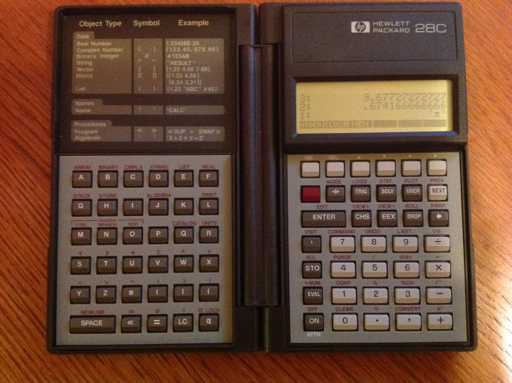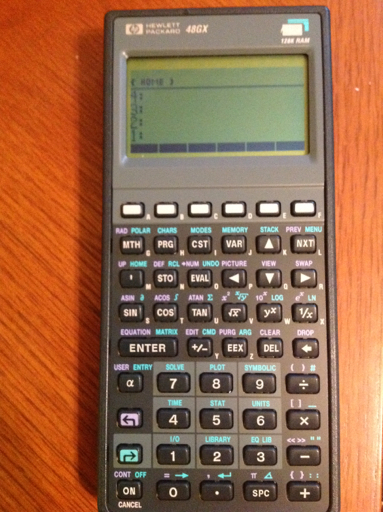## Tuesday, June 19, 2012

### Casio Programming Part II

This blog entry is an extension of the list of programs made with the Casio fx-6300G and the fx-7000GA. Most of the program steps can be transferred verbatim to today's Casio graphing calculators.

Note:
Pol( and Rec( on the fx-6300G and fx-7000GA returns results in variables I and J. I gets r and x, respectively while J gets y and θ, respectively.

The fx-9xxx series (at least the fx-9860 series) returns the conversions from Pol( and Rec( as lists. Adjust accordingly.

However this list of programs will bring more of the complex features offered by today's higher-end calculators.

Here are the links to my two previous posts:

Casio and the Programming Language

Program Library Part 1

The batteries run out on the fx-7000GA fast, or maybe not. It seems to work well after hours of rest.

The programs listed (except for the Quadratic Formula program) on this blog requires a second program, which I designated Program 0. I use program 0 to store my function.

Calculus

Numeric Derivative Approximation

The difference quotient method is used with Δ = 10^-5. Accuracy may vary. Machine accuracy not guaranteed.

Program 0: Enter as a function of X. (example: "2 sin x")

D is the derivative.

Main Program:
"X0"?→Z:
Z + 10^x -5→X: Prog 0: Ans→A:
Z - 10^x -5 → X: Prog 0: Ans→B:
(A - B) ÷ (2 10^x -5) → D

51 steps

Sum of a Function

Σ f(x) from a to b

S is the sum

Program 0: Function of X

Main Program:
"A"?→A:"B"?→B:
A→X: 0→S: Lbl 1:
Prog 0:Ans + S → S:
X + 1 →X:X ≤ B ⇒ Goto 1: S

48 steps

Simpson's Rule - Numeric Integration

I modified the program in the Casio fx-6300G handbook, to try to make it shorter.

N must be even. I is the integral.

Program 0: Function of X

Main Program:
"A"?→ A: "B"?→ B:
"N"?→ N: A→ X: Prog 0: Ans → I:
(B - A) ÷ N → D: N- 2 → O:
Lbl 2:
X + D → X: Prog 0: I + 4 Ans → I:
X + D → X: Prog 0: I + 2 Ans → I:
O - 1 → O: O ≠ 0 ⇒ Goto 2 :
B → X : Prog 0 : (I - Ans) D ÷ 3 → I

113 steps

Graphing: Parametric Equations

Program 0: X(T) to be stored in X, Y(T) to be stored in Y
(Example: 2T sin T→ X: 3T cos T→ Y)

Note: You will have set up the Range in advance which takes intuition, knowledge, and maybe luck to get a "perfect" graph. Once the Range is set up, as I understand it, the graph screen clears.

A = Tmin
B = Tmax
N = number of points

Main Program:
"A"?→A: "B"?→ B:
"N"→ N: (B - A) ÷ N → H: Cls : A - H → T:
Lbl 1: T + H →T:Prog 0: Plot X,Y : T ≠ B ⇒ Goto 1

61 steps

Graphing: Polar Equations

Program 0: R(T) with T being θ
(Example: .5T)

Note: You will have set up the Range in advance which takes intuition, knowledge, and maybe luck to get a "perfect" graph. Once the Range is set up, the graph screen clears.

A = Tmin
B = Tmax
N = number of points

I had better luck with this program on the fx-6300G than on the fx-7000GA.

Main Program:
"A"?→A: "B"?→ B:
"N"→ N: (B - A) ÷ N → H: Cls : A - H → T:
Lbl 1: T + H →T:Prog 0: Rec(Ans,T: Plot I,J : T ≠ B ⇒ Goto 1

67 steps

Note: these are dot plots and are not traceable. I tried using SD2 mode (Scatterplot Mode) but was not able to use comparison commands. Also Line is not the command that it is today.

And now to a favorite program...

AX^2 + BX + C = 0

D = B^2 - 4AC

If D <0, then the roots are complex, X is the real part, Y is the imaginary part, X ± Yi
If D >0, then the roots are X and Y, both real

Program displays D, X, and Y

Preload A, B, and C before running!

B^2 - 4AC → D ◢
D < 0 ⇒ Goto 1:
(-B + √ D) ÷ (2A) → X:
(-B - √ D) ÷ (2A) → Y: Goto 2:
Lbl 1: -B ÷ (2A) → X : √ Abs D ÷ (2A) → Y :
Lbl 2 : X ◢ Y

79 steps

I leave you with some graphs.

Until next time, Eddie

This blog is property of Edward Shore. © 2012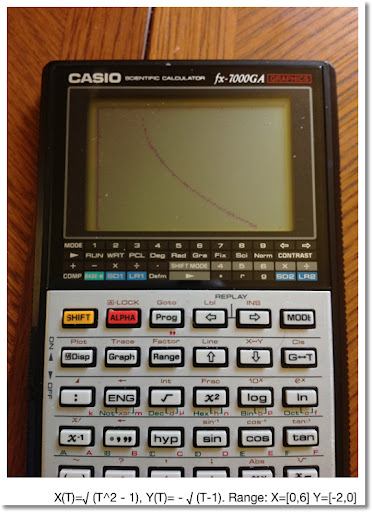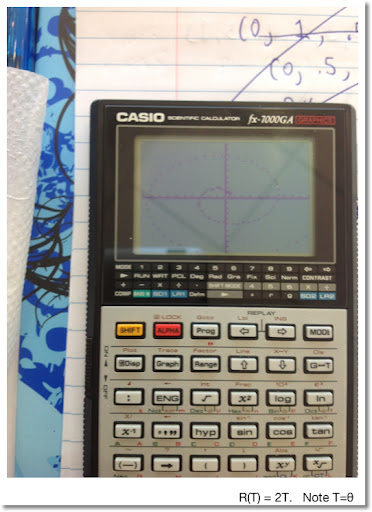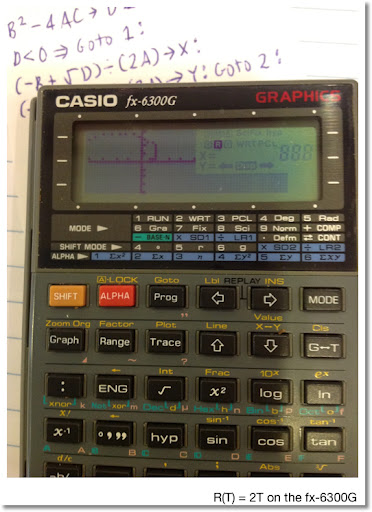## Sunday, June 17, 2012

### Casio Program Library Part 1

Here is a list of programs I did over the course of the last few days (since 6/14/2012). These programs were done using the Casio fx-6300G and fx-7000GA calculators. These programs can be ported to almost any Casio calculator that has programming.

Note: for the fx-3650P, there are no strings, graphics, and variables are limited to A, B, C, D, X, Y, and M.

If you need summary of the programming commands, check out this page.

Programs are listed in blue, Courier font. Enjoy! Eddie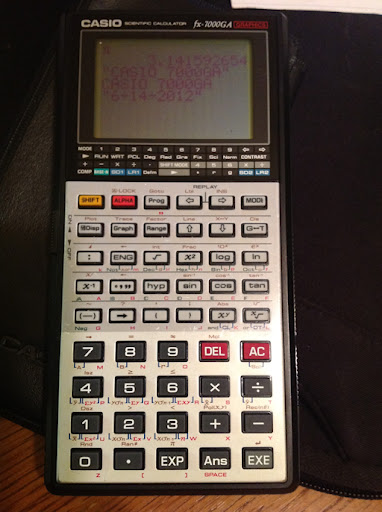Binomial Distribution

B
Σ N nCr K × P^K × (1-P)^(N-K) = M
K=A

A = lower limit
B = upper limit
P = probability of success (0 ≤ P ≤ 1)
M = total probability

For a single point, enter A and B as the same value

**You can shave off steps by pre-storing all the variables before execution and leaving out some the beginning prompt commands. I do that in some of these programs.

"A"?→A:"B"?→B:"N"?→N:"P"?→P:
0→M:Lbl 1:
M+(N!×P^A×(1-P)^(N-A))÷(A!(N-A)!)→M:
A+1→A:A>B⇒Goto 2:Goto 1:
Lbl 2:M

91 steps

Roots of a Complex Number

Uses the polar form of the complex number x+yi to find the n roots of it.

Note: fx-6300G and fx-7000G Rectangular and Polar Conversions

Pol(x,y) stores r in I and θ in J.
Rec(r,θ) store x in I and y in J.

For the fx-9000s series (i.e. fx-9860), lists are returned. Adjust accordingly.

(x+yi)^1/n = r^1/n cos((θ + 2k π)/n) + i r^1/n sin((θ + 2k)/n)

"X"?→X:"Y"?→Y:"N"?→N:
Pol(X,Y:Lbl 1:"RE"◢
N x√ I × cos((J+2K π ) ÷ N ◢
"IM" ◢ N x√ I sin((J+2K π ) ÷ N ◢
1 + K → K ◢ K < N ⇒ Goto 1

89 steps

Horizontal Curves

L = R θ
C = 2 R sin(θ/2)

where L=length of arc and C=chord length

Let T be θ.

"R"?→R: "T"?→T:
"C" ◢ 2R sin(T ÷ 2

34 steps

Lens Equation: Image Length and Magnitude

O^-1 +I^-1 = F^-1
M = F (F-O)^-1

Where
O = object length
F = focal length
I = image length
M = magnification

Notes:

Focal Length
F<0 for concave lens and convex mirrors (diverging light)
F>0 for convex lens and concave mirrors (converging light)

Magnification
M>0 image is upright
M<0 image is upside down
0<|M|<1 image is shrunk
|M|>1 image is enlarged

"O"?→O: "F"?→F:
"I" ◢ (F^-1 - O^-1)^-1 ◢
"M" ◢ F(F-O)^-1

38 bytes

Ellipse

With semi-axis of lengths X and Y:

Area = π X Y

Perimeter ≈ 2 π √ ((X^2 + Y^2) / 2)
(best when X and Y are close, this came from the Fundamentals of Engineering Handbook)

"X"→ X: "Y"→ Y:
"P" ◢ 2 π √ (.5 (X^2+Y^2→ P ◢
"A" ◢ π XY → A

42 steps

2 by 2 Matrices: Determinant, Inverse, Solution

[[A B][C D]] [[X][Y]] = [[E][F]]

inverse = [[D/Z -B/Z][-C/Z A/Z]] = [[G H][I J]]

solution = M^-1 b = [[G H][I J]] [[E][F]] = [[X][Y]]

If Z = 0, the matrix is singular and only the determinant is displayed.

Store A, B, C, D, E, and F before running the program

"DET" ◢
AD-BC→Z ◢ Z = 0 : Goto 1 : "INV" ◢
D ÷ Z → G ◢ -B ÷ Z → H ◢ -C ÷ Z → I ◢
A ÷ Z → J ◢ "X,Y" ◢ GE + HF → X ◢
IE + JF → Y

74 steps

Picking Random Numbers - Repeats are Allowed

N random numbers from A to B, with repeats allowed

"A"?-> A: "B"? → B:
"N"?→ N: Lbl 1: Int((B-A+1)Ran#) ◢ Dsz N : Goto 1

40 steps

There will be a Part 2 - which I will aim to post during the next week. Until then,

Eddie

This blog is property of Edward Shore. © 2012

### Casio fx-7000GA and the Programming Language

Greetings all!

I finally got my hands on it! The Casio fx-7000GA calculator. OK, it is not the original fx-7000G that launched the world of graphing calculators, but it is the second edition of it. The 7000G was originally launched in 1985. After some research, i found that the fx-7000GA was first launched in 1990. The fx-7000G series continued with the fx-7000GB (1992) and the fx-7200g (1986). This series is like the Texas Instruments TI-83/84+ family (1996-present), except that the latter family eventually added flash ROM and textbook math input on its later models.

Why?

I spent a week playing with the Casio fx-3650P, a solar programming Calcualtor that is sold today (had to buy one off of eBay). And being a collector, the fx-7000G is on my wish list.

An excellent page on programming calculators: http://www.rskey.org/CMS/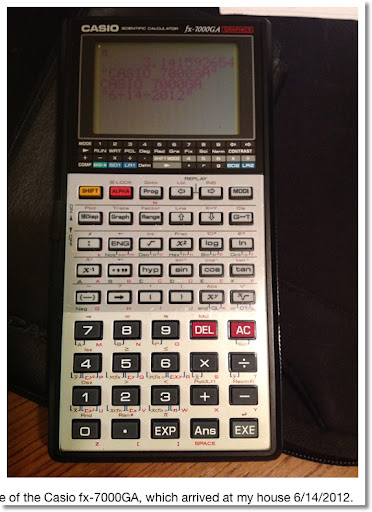The fx-7000GA arrived at my house on 6/14/2012. I was bummed at first because the calculator acted weird. Thankfully, three fresh CR2032 batteries later, the calculator is back in working order. However I read that the batteries get used quickly. Bummer.

I am amazed on how light the fx-7000GA is. I was expecting a heavy machine, being one of the first graphing calculators to ever be mass produced. However the calculator is thin, which hopefully you can see in a picture comparing the fx-7000GA and the TI-84+ below.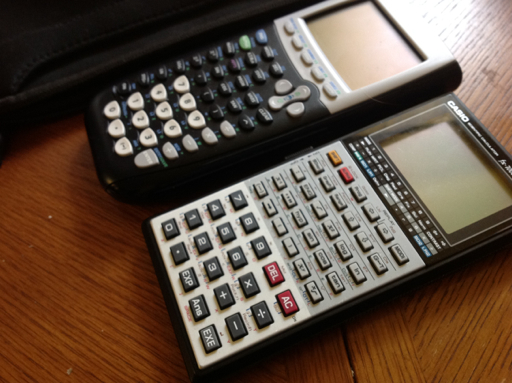Features of the FX-7000GA

Graph Types: Function, Scatterplot, Histogram
Memories: 26, but can be expanded to 78
Program Steps: 422, 10 program slots
Linear Regression
Base Operations: Decimal, hexadecimal, octal, binary

The fx-7000G's smaller cousin, the fx-6300G, which I got several years back in Hollywood, CA (new of the box!), has only 400 program steps but adds the XNOR function. The fx-6300G has a lot of clones.

Programming Language

The programming language is a simple, tokenized one. Tokenized means that symbols and characters are used instead of words to symbolize commands. This minimizes the memory used in programs. This type of programming language is prevalent in today's graphing calculators from Casio. It also requires us bloggers to purchase and use Unicode apps to get these symbols.

The commands featured are:

: This colon separates commands.

? Input Command. The calculator prompts for a value.

"prompt message"? Input Command with a prompt message.

◢ Output Command. The calculator stops and shows output. Continue the program by pressing EXE. On the fx-3650P and fx-6300G, when ◢ is encounter during program execution, the calculator stops and a Disp indicator is present.

Goto n The Goto command, n is any number 0-9.

Lbl n The Label command, n is any number 0-9.

⇒ The Jump command. This is the basic If-Then-Else structure. On the fx-7000G and fx-6300G, the command is entered by pressing SHIFT, 7.

Syntax:

test statementdo if test is true : skip to here if test is false

Isz and Dsz Increase and decrease a memory, by 1, respectively. This is akin to the For-Next loops.

Syntax:

Isz var : do if var ≠ 0 : skip to here if var=0

Syntax is the same for Dsz.

My next blog entry will have some of the programs I entered over the last few days since I got the fx-7000GA. Until then, Cheers! Eddie

This blog is property of Edward Shore. © 2012

## Sunday, June 10, 2012

### My Favorite Solar Calculators of all time

In no particular order...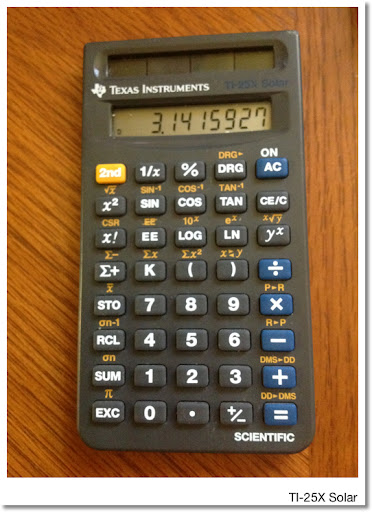Texas Instruments TI-25X Solar - mid to late 1990s

This small calculator packs a lot of punch. 8 digit that converts to 5 digit with 2 digit exponent any time the number exceed eight digits. Features include reciprocal, polar/rectangular conversion, one variable statistics, and decimal-DMS conversion. When I had the TI-25X the first time, back in the late 90s, it was my go-to calculator for quick calculations. I just bought another one from Amazon a week ago.

The calculator is truly solar, that is no batteries are used in any operation, which is rare in solar scientific calculators. (The battery, I think, aids long-term memory storage).

The trouble with the 25X was protecting it. It now lives in a small camera case with bubble wrap around it when not in use. I wish Texas Instruments was still manufacturing the 25X.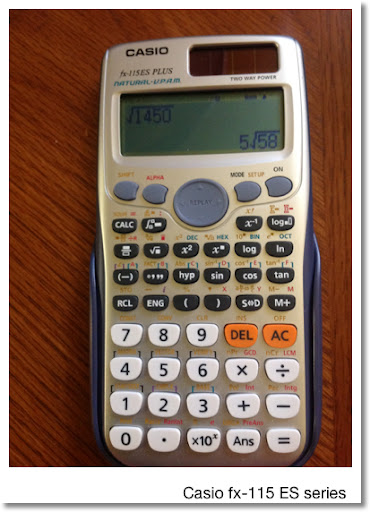Casio FX-115ES Series - 2004-present

This is my favorite calculator Casio has produced. Not only the calculator operates on solar power, but it also was the first (I believe) calculator to have calculus functions: integral, derivative, and sum of a function. Matrices and vectors are also featured. The calculator can solve equations in one variable. Entry of mathematical expressions is straight forward. You can set the calculator to textbook entry mode or linear (classic) mode. I prefer the latter (the less pairs of parentheses I have to track the better).

The 2012 revision, fx-115ES PLUS (pictured), adds, among other things, integer factoring, verification tests, and product of a function.

Outside the United States, this calculator is named the fx-991DE/ES (PLUS).

Texas Instruments BA 35 Solar - early 1990s
(not pictured :( - I don't have it anymore)

Anyone who can operate a BA 35 or a BA II Plus should feel comfortable with the BA 35 Solar. The BA 35 Solar has the following:

* Time Value of Money, using periodic interest rate.
* Cost Sell Margin/Markup calculations
* Interest Conversion
* Amortization
* One variable statistics.

I gave my BA 35 Solar to my dad and he loved using it. Unfortunately, the display went nuts and it had to be retired.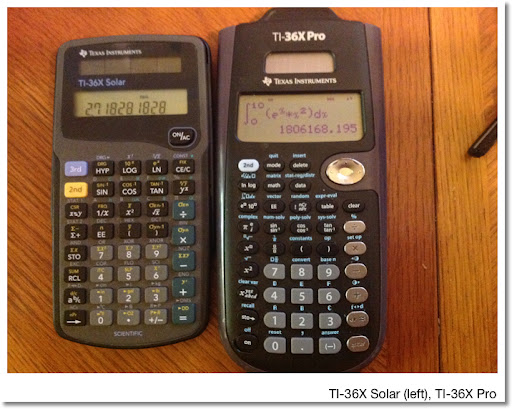Texas Instruments TI-36X Series (TI-36 Solar: 1986-early 1990s, TI-36X Solar: 1993-present, TI-36X Pro: 2011-present)

If you wanted the most advanced non-graphing calculator, you pretty much went for the TI-36X series. The original TI-36 Solar was powerful non-programmable calculator which had base conversions, hyperbolic functions, normal distribution calculations, and complex numbers (limited to arithmetic). When Texas Instruments upgraded the 36 to the 36X, the normal distribution calculations and complex numbers were dropped, but eight scientific constants, eight metric-English conversions, and linear regression were added.

This calculator received a lot of use during my days of middle and high school. I owned the original 1993 design and it went with me everywhere I went. The solar panel was punctured by a pencil and it died. Eventually, I got the 1996 design (pictured) and is my favorite design of the TI-36X Solar. Texas Instruments redesigned the calculator in 2004, giving it a gray faceplate and white keys, which I also have.

In 2011, Texas Instruments released the TI-36X Pro (named TI-30X Pro in Europe), which a serious upgrade of the 36X series. New features include derivatives, integrals, tables, equation solver, and entry in textbook format. For me, I had to get used to the keyboard because a lot of the keys cycled through functions. An example: the sine key (sin, sin^-1, sinh, sinh^-1), math constants ( π , e, the complex number i ), and the key that annoys me the most, the variable key (x, y, z, t, a, b, c, d).

If you waited to 2012 to consider getting a TI-36X Pro, you did a good thing in waiting. The early versions were buggy and were erroneous in calculations involving π and fractions. Thankfully, TI fixed the bugs and now I can confidently use the TI-36X Pro.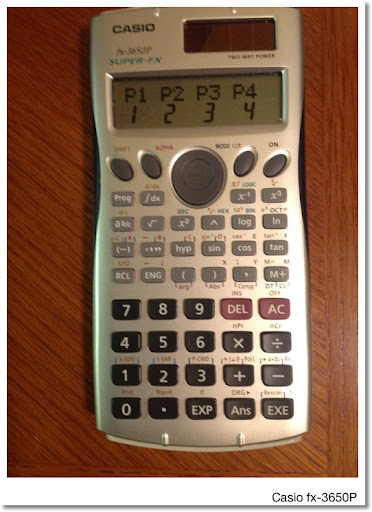Casio fx-3650P: 2002 - present?

Casio, I think, is the only manufacturer of calculators left that produces programming calculators that also operate on solar power (with battery). I purchased this calculator on eBay (unfortunately it is not sold in stores in the United States, it should be IMHO).

The calculator has 360 program steps on which 4 programs can be stored. The programming language is a simplified set of the programming commands found on the Casio fx-5800p and its graphing calculators. Commands include LABEL, GOTO, basic testing, input prompt, and output.

Other features include complex number arithmetic, base conversions, and Numeric integrals.Victor V34 - 2000s

Take a good loom at this calculator. If this calculator reminds you of the super popular Texas Instruments TI-30X IIs, it is because the keyboard design is similar.

While the TI-30X IIs sold in many colors for the 2009, 2010, 2011, and (I'm expecting) 2012 back to school seasons, the Victor V34 offers a feature that the TI-30X IIs doesn't: base conversions and logic operations. I purchased this hard to find calculator in a local stationery store in Glendora, CA.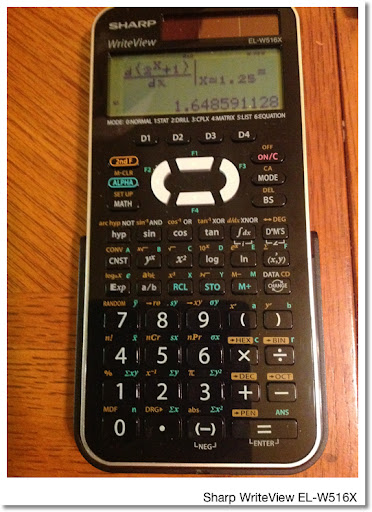Sharp EL-W516 Series - 2000s - Present

There are two functions two versions. The original had a gray faceplate. Sometime in 2011(?), Sharp made a design change and made the entire plate black. The model is now the EL-W516X with black keys, white, green, and gold lettering. In the United States, the Sharp calculators can be found at Target stores.

Like the Casio fx-115 ES, the Sharp EL-W516 series offers entering calculations in textbook format. Unlike the fx-115ES where you can get exact or approximate answers when demanded, the EL-W516 forces you to cycle through the format of the answers (exact/mixed fraction, improper fraction, decimal approximation) with the press of the CHANGE key (this applies to Normal mode).

The Sharp EL-W516 series offers unique functions:
* Drill feature - a quiz of arithmetic problems
* Functions with lists
* 4 definable function keys
* 4 definable formulas (I love this feature)
* Pental Numbers (Base 5)

That is a list of my favorite solar calculators. Eddie

This blog is property of Edward Shore. © 2012

## Wednesday, June 6, 2012

### Arithmetic, Powers, Percent Change, and Square Roots using the Printing Calculator

Printing calcualtors, better known as adding machines and 10-key machines are calculators designed for the office. If you are a professional accountant, CEO, auditor, tax preparer, or supervisor, chances are you operate these machines at least once in a while if not every day. The Sharp EL-1750V printing calculator is pictured below.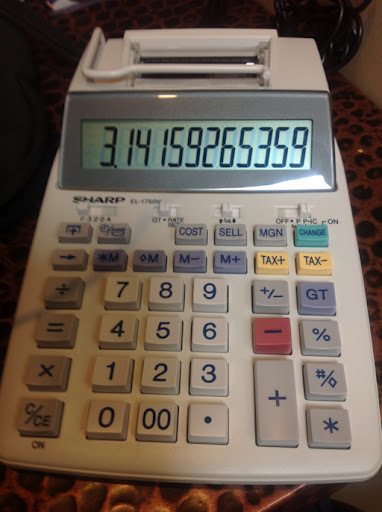Introduction

This blog entry will show you how to extend the operation of the printing calculator beyond the mere adding and subtracting. While a printing calculator may not be a weapon of choice for super advanced mathematics, we can use some of the features to do some cool arithmetic and algebraic problems. Amaze your office friends with these techniques!

Note: I will assume the calculator is set to Float (F) mode and not in Add mode (A, or +)

The Printing Calculator

You have a printing office calculator if you have keys such as +=, -=, *T, ♢S, and GT.

The printing calculator operates on a hybrid notation. Addition and subtraction operate by postfix notation. That is, enter the number, then press the + key or - key, and repeat. Addition and subtraction operations are terminated by a totals key, labeled *, *T, or */T. For this blog entry, I will use *T to symbolize the totals key.

Example 1: 45 + 68 + 99 - 100 = 112

Keystrokes will be in blue and Courier font.

45 +
68 +
99 +
100 - *T

Multiplication and division operate by infix notation. This is enter the first number, press either the × or the ÷ key, then enter the second number. Multiplying and dividing operations are terminated by the equals key. The equals key can be by itself (=) or coupled by a plus or minus operation (+= or -=).

Example 2: 24 × 72 ÷ 6 = 288

24 ×
72 ÷
6 =

The memory keys are your good friends on the printing calculator. Here is a basic rundown of the memory keys:

M+: Adds whatever is in the display to the memory register
M-: Subtracts whatever is in the display from the memory register
MS or M♢ : Displays and recalls the contents of the memory register
MT or M* : Recalls the contents of the memory register AND resets the memory register to 0.

For this blog entry, I will use the M ♢ and M* notation. It is often a very good idea to "clear memory" first by pressing M* before doing several of the more complex calculations.

Now that you have it, let's go on to the arithmetic calculations. Paper printing is optional but unless you have ton of tape, it is not really necessary.

Arithmetic

Couple of Golden Rules:

1. Make sure memory is clear before beginning (necessary most of the time).
2. If you remember "My Dear Aunt Sally" (multiplication and division before adding and subtracting), the rules of parenthesis, and the rules involving rational expressions, we're in business. See, algebra class pays off!
3. Do not forget to terminate adding/subtracting calculations with *T before switching to multiplying/dividing.
4. Likewise, do not forget to terminate multiplying/dividing calculations with = before switching to adding/subtracting. Before starting the adding/subtracting, press the + key once to tell the printing calculator to add the number.

Let's begin. I use the capital letters A, B, C, etc. to represent numbers (as variables).

#1. A × B + C
Keystrokes:
A × B =
+ C + *T

Example: 5 × 2 + 10 = 22
5 × 2 =
+ 12 + *T

#2. (A + B) ÷ C
Keystrokes:
A + B + *T
÷ C =

Example: (56 + 27) ÷ 3 = 27.6666666667
56 + 27 + *T
÷ 3 =

Here is where the memory keys get used. If you have to switch signs (i.e. negate a value), remember to use the plus/minus key +/- .

#3. A × B + C × D
Keystrokes:
M*
A × B = M+
C × D = M+
M*

Example 1: 4 × 1.99 + 8 × .99 = 15.88
M*
4 × 1.99 = M+
8 × .99 = M+
M*

Example 2: A bill with sales tax.
(2 × 19.95 + 10.99 + 3 × 4.99) + 8%
= (2 × 19.95 + 10.99 + 3 × 4.99) × (1 + 8 ÷ 100)
= (2 × 19.95 + 10.99 + 3 × 4.99) × 1.08
M*
2 × 19.95 = M+
10.99 M+
3 × 4.99 = M+
M* × 1.08 =

#4. A × B - C × D
Keystrokes:
M*
A × B = M+
C × D = M-
M*

Example: 3 × 4 - 2 × 3.1 = 5.8
M*
3 × 4 = M+
2 × 3.1 = M-
M*

#5. (A + B) ÷ (C + D)
The trick here is to work on the denominator first. Keystrokes:
M*
C + D + *T M+
A + B + *T
÷ M* =

Example 1: (6 + 3.9) ÷ (5 - 4.1)
M*
5 + 4.1 - *T M+
6 + 3.9 + *T
÷ M* =

Example 2: 2 × (3 + 4) ÷ (7 + 8) = .93333333333
M*
7 + 8 + *T M+
3 + 4 + *T
÷ M*
× 2 =

#6: Working With Mixed Fractions
Mixed fractions in the form of A B/C can be entered with the following keystrokes:
B ÷ C = + A + *T

Be sure to work with memory when you adding and subtracting fractions. You will get an answer in decimal format, no matter how nice your fractions are.

Example: 4 2/5 + 3/7 - 2 1/9 = 2 226/355 = 2.71746031746
M*
2 ÷ 5 = + 4 + *T M+
3 ÷ 7 = M+
1 ÷ 9 = + 2 + *T M-
M*

Cost/Sell/Margin and using those keys to find Δ%

#7. Margin
The equation for Cost/Sell/Margin calculations is:

100 × (sell - cost) ÷ sell = margin

Let A be the cost of the item and B be the selling price.
Keystrokes:
A COST
B SELL (Margin is automatically calculated)

Example: If the cost of an item is \$3,200 and the selling price is \$4,800, what is the margin?
3200 COST
4800 SELL

The margin is 33.3333333333%

#8. Percent Change Δ%
The equation for percent change is:

100 × (new - old) ÷ old = Δ%

Note that

100 × -1 × (old - new) ÷ old = Δ%

It looks like the Cost/Sell/Margin equation above. We can use the Cost/Sell/Margin and the change sign (+/-) to calculate Δ%.

Let A be the old number and B be the new number.
A SELL
B COST +/-

Example: Find the percent change from 17.95 to 24.65. 17.95 is the old number, 24.65 is the new number.
17.95 SELL
24.65 COST +/-

Δ% = 37.3259052924%

Reciprocals and Powers

#9. Reciprocals

The reciprocal of A is 1 ÷ A.

We can use straight division, memory, or a third technique which can be useful in more complex calculations.
A ÷ = =

Example 1. Calculate the reciprocal of 4. (4^-1)
4 ÷ ÷ =

4^-1 = 1 ÷ 4 = 2.5

Example 2. 1 ÷ (60.7 - 38.6) = (60.7 - 38.6)^-1 = .0454886877
60.7 + 38.6 - *T ÷ ÷ =

#10. Powers

To raise a number A to a power N (N is an integer), use the following technique:
1. Enter A
2. Press the × key N-1 times.
3. Press the = key.

To square A, press A × =.
To cube A, press A × × =.

For practical purposes, N should not be too high, unless you want to press × many times while keeping a mental count.

If N is negative, follow the power calculation with a reciprocal calculation. See Example 2.

Example 1: 3.6^3 = 46.656
3.6 × × =

Example 2: 5000 × 1.1^-4 = 3,415.50672768
1.1 × × × =
÷ ÷ =
× 5000 =

Example 3: (3 + 2.4)^2 - (3.1 - 2.8)^3 = 29.133
*M. (clear memory)
3 + 2.4 + *T × = M+
3.1 + 2.8 - *T × × = M-
*M

Square Root Routine

One of the most annoying things about printing calculators is the lack of a square root key (√). There is an iterative method for finding the square root using the Babylonian Method (if you want a challenge or don't feel like getting a calculator with a square root function or running Excel):

To find √S (the square root of S):

1. Start with an initial guess x0. Obviously, the better the initial guess, the faster this method goes.
2. Calculate x1 = (x0 + S ÷ x0) ÷ 2
3. As you repeat Step 2, more and more decimal places should "repeat" themselves. For each loop, set x1 as the new x0.

Keystroke wise:
To start: M* x0
Loop: M+ S ÷ M♢ = M+ M* ÷ 2 =

Example: √125 with an initial guess x0=10
M* 10

Loop #1:
M+ 125 ÷ M♢ = M+ M* ÷ 2 = (11.25)

Loop #2:
M+ 125 ÷ M♢ = M+ M* ÷ 2 = (11.1805555555)

Loop #3:
M+ 125 ÷ M♢ = M+ M* ÷ 2 = (11.1803398895)

Loop #4:
M+ 125 ÷ M♢ = M+ M* ÷ 2 = (11.1803398874)

Loop #5:
M+ 125 ÷ M♢ = M+ M* ÷ 2 = (11.1803398874). STOP

Hence √125 = 11.1803398874

Have fun impressing your co-workers and friends. It's been a blast as always! Until next time, Eddie

This blog is property of Edward Shore. © 2012

## Sunday, June 3, 2012

### New Videos: Leases

Regular Leases

Leases with Payments Required in Advance

An awesome page about leases, thanks tvmcalcs.com!

Hope this helps! Eddie

This blog is property of Edward Shore. © 2012

### Fun With the HP 71B

This blog entry is dedicated to Namir Shammas, who just became a grandfather.

Greetings everyone! Recently I spent a week programming on an absolute beauty: the HP 71B calculator from Hewlett Packard. The calculator was manufactured during the mid 1980s, has a memory of over 16,000 bytes and four slots for software pacs or ROM PACs (cartridges). Currently I do not have any cartridges for the 71B at this time.

One cartridge gives the user access to the FORTH programming language on the 71B, which allows for the 71B to operate in RPN (Reverse Polish Notation) mode. Here is a video by AshLeaFen on 41 Translator ROM PAC on the 71B.

I won this calculator as a door prize, believe it or not, at a calculator convention last year.

BASIC PROGRAMMING

The primary feature of the HP 71B is its programming. The 71B has a QWERTY keyboard, which is just a pleasure to type on. The calculator's programming is versatile. To give a couple of examples:

Assigning a variable: I could use either a LET statement or just use the equals key by itself.

10 LET A = 1

or

10 A =1

The IF-THEN-ELSE structure. If there is just an integer after the THEN or ELSE, the calculator goes to that line without the need for a GOTO command. So:

50 IF X=1 THEN 100 ELSE 200

is just as effective as

50 IF X=1 THEN GOTO 100 ELSE 200

This trick works with line numbers only as I understand it.

I think preceding every line with line numbers is a lost art. While there may no longer be a need for them in today's languages, line numbers do give an order or structure to the program. The HP 33S and 35S programming calculators (also made by Hewlett Packard) keeps this tradition alive, except that line numbers are structured.

Below the picture of it are several of the programs I made over the last week. When it is not used, the 71B is stored in a Kindle case.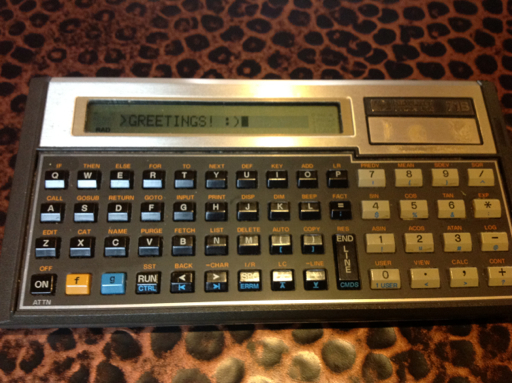LIST OF PROGRAMS

PROGRAM CTDWN (Countdown Timer)
11/16/2011 150 Bytes

10 DESTROY I
20 INPUT "# of seconds:";I
30 ON TIMER #1,1 GOSUB 60
40 DISP "Tick... Tock..."
50 IF I=1 THEN 90 ELSE 40
60 I=I-1
70 DISP I
80 RETURN
90 OFF TIMER #1 @ DISP I
100 WAIT I
110 DISP "Time's Up!"
120 END

This program uses the 71B's internal clock to make a countdown timer.

PROGRAM LIST1 (list of random integers)
158 bytes, 5/28/2012

10 OPTION BASE 1
20 DESTROY L
30 RANDOMIZE
40 INPUT "LENGTH? ";K
50 DIM L(K)
60 INPUT "HIGH NUMBER? "; N
70 FOR I=1 TO K
71 BEEP 512,.5
72 L(I)=IP(N*RND+1)
74 DISP "L(";I;")=";L(I)
76 WAIT 2
78 NEXT I
80 DISP "Done, check L."

The program generates a list of random integers from 1 to N. I have the 71B set the random seed to current time. Since this program leaves L as an array, if you want to use L as another variable, you probably have to execute DESTROY L first.

PROGRAM CARTH (Complex Number Arithmetic)
253 Bytes, 5/29/2012

10 DISP 'Complex Arithmetic'
20 INPUT "A,B(i),C,D(i):", A,B,C,D
30 DISP '1. + 2. - 3. * 4. /' @ WAIT 1.5
40 INPUT "Choice?"; H
50 ON H GOTO 60,70,80,90
60 DISP A+C;'+i';B+D @ END
70 DISP A-C;'+i';B-D @ END
80 DISP A*C-B*D;'+i';B*C+A*D @ END
90 DISP (A*C+B*D)/(C^2+D^2);'+i';(B*C-A*D)/(C^2+D^2) @ END

This is your basic arithmetic with complex numbers. The ON variable GOTO structure allows you to assign various GOTO commands in one line based on the value of variable.

PROGRAM RWALK (Random Walk of 50 Steps)
131 bytes; 5/30/12 - 50 steps - θ g CTRL P

10 DISP 'Random Walk'
15 DESTROY T,N,I
20 I=10 ! TAB POSITION
30 RANDOMIZE
40 FOR I=1 TO 50
42 N=IP(3*RND)-1
44 T=T+N
46 DISP TAB(T); 'θ'
48 NEXT I
50 WAIT 1
52 DISP 'Position:'; T

Where will the out of control θ end up?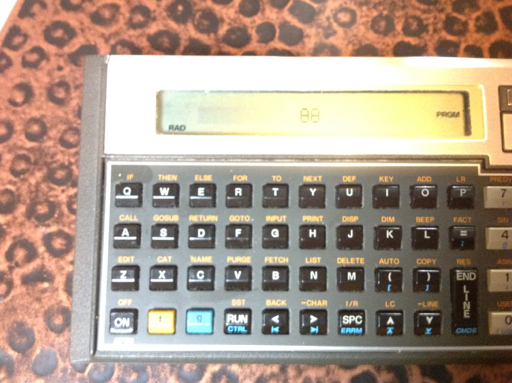PROGRAM MATRIX1
238 Bytes, 11/16/2011

10 DESTROY A,B,C,D,E,A1,B1,C1,D1
20 INPUT "[[A,B][C,D]]:";A,B,C,D
30 E=A*D-B*C
40 DISP "DETERMINANT=";E
50 PAUSE
60 IF E=0 THEN STOP
70 A1=A/E @ B1=-B/E @ C1=-C/E @ D=D/E
80 DISP "INV="
90 WAIT 1
100 DISP "I11=";D1 @ WAIT 2
110 DISP "I12=";B1 @ WAIT 2
120 DISP "I21=";C1 @ WAIT 2
130 DISP "I22=";A1 @ WAIT 2
140 END

This program gives the determinant, and if it exists (det M ≠ 0), the inverse of a 2x2 matrix.

PROGRAM HORIZ (Horizontal Curve)
145 Bytes, 5/26/2012

100 DISP 'Horizontal Curves'
120 INPUT "Angle (DEG)? ";A
130 DEGREES
140 LET C=2*R*SIN(A/2)
150 DISP 'Chord Length=';C
155 PAUSE
170 DISP 'Chord Length=';L

A program for horizontal curves (engineering).

PROGRAM SUMFX (Σ f(x) a to b)
100+ bytes, 5/27/2012

Edit f(X) at line 10

10 DEF FNF(X)=insert function of X here
20 INPUT "Lower=";L
30 INPUT "Upper=";U
40 T=0
50 FOR X=L TO U @ T=T+FNF(X) @ NEXT X
60 DISP 'Total=';T

PROGRAM DERVX
100+ bytes, 5/27/2012

10 DEF FNF(X)=insert function of X here
20 INPUT "VALUE=";V
30 INPUT "TOLER 10^-";H
35 H=10^(-H)
40 D=(FNF(V+H)-FNF(V-H))/(2*H)
50 DISP "d/dX=";D

These programs calculate the sum of a function and the numeric derivative of a function, respectively. Line 10 is where you have to define your function (of X).

That concludes my blog entry for today.

P.S. Hewlett Packard, when will the HP 39gii calculator be on sale in the United States? Thanks!

This blog is property of Edward Shore. © 2012

### HP 42S/DM42: Height of a Fire, Time to Clear a Corridor

HP 42S/DM42:  Height of a Fire, Time to Clear a Corridor HP 42S/DM42/Free42 Program:  FIREHGT The program FHGT approximates the height of a...